• 2019-08-16 18:24:43

# 学习前言

刚刚从大学毕业，近来闲来无事，开始了机器学习的旅程，深度学习是机器学习的重要一环，其可以使得机器自我尝试，并通过结果进行学习。在机器学习的过程中，我自网上了解到大神morvanzhou，一个从土木工程转向了计算机的“聪明绝顶”的、英语特好的男人。
morvanzhou的python个人主页，请有兴趣的同学关注大神morvanzhou的python教程。

## 简介

我们使用神经网络输入当前的状态，网络就可以输出我们在这个状态下采取每个动作的概率。
与之前的DQN和Q-Learmnig这类基于价值的学习方法相比，二者的主要不同为：
2、DQN之类的方法一般都是只处理离散动作，无法处理连续动作。

## 举例应用

本文使用了OpenAI Gym中的CartPole-v0游戏来验证算法的实用性。
该环境只有两个离散动作，板子可以向左滑动或者向右滑动，保持杆子不倒即可。
state状态就是这个板子的位置和速度， pole的角度和角速度，4维的特征。

### 神经网络的构建

其建立的神经网络结构如下：
神经网络的输入量是self.tf_obs，指的是环境的状态。
神经网络的输出量是self.all_act_prob，指的是每个动作的概率。
神经网络共两层。

def _build_net(self):
# 输入量
# self.tf_obs指的是环境的观测量
# self.tf_acts指的是可执行的动作
# self.tf_vt指的是每个动作的奖惩情况
with tf.name_scope('inputs'):
self.tf_obs = tf.placeholder(tf.float32, [None, self.n_features], name="observations")
self.tf_acts = tf.placeholder(tf.int32, [None, ], name="actions_num")
self.tf_vt = tf.placeholder(tf.float32, [None, ], name="actions_value")

# 利用tf.layers.dense函数生成全连接层fc1
layer = tf.layers.dense(
inputs=self.tf_obs,
units=10,
activation=tf.nn.tanh,  # tanh activation
kernel_initializer=tf.random_normal_initializer(mean=0, stddev=0.3),
bias_initializer=tf.constant_initializer(0.1),
name='fc1'
)
# 利用tf.layers.dense函数生成全连接层fc2
all_act = tf.layers.dense(
inputs=layer,
units=self.n_actions,
activation=None,
kernel_initializer=tf.random_normal_initializer(mean=0, stddev=0.3),
bias_initializer=tf.constant_initializer(0.1),
name='fc2'
)

self.all_act_prob = tf.nn.softmax(all_act, name='act_prob')  # use softmax to convert to probability

with tf.name_scope('loss'):
# 可以通过最小化-(log_p * R)的方式实现最大化(log_p * R)
neg_log_prob = tf.nn.sparse_softmax_cross_entropy_with_logits(logits=all_act, labels=self.tf_acts)
loss = tf.reduce_mean(neg_log_prob * self.tf_vt)  # reward guided loss

with tf.name_scope('train'):


### 动作的选择

其根据神经网络的输出按照概率选择动作。

def choose_action(self, observation):
prob_weights = self.sess.run(self.all_act_prob, feed_dict={self.tf_obs: observation[np.newaxis, :]})
action = np.random.choice(range(prob_weights.shape), p=prob_weights.ravel())
return action


### 神经网络的学习

神经网络的学习过程是这样的，通过记录每一轮开始直到done结束的所有的状态、动作及其得分。
通过所有的状态得到所有状态下每个动作的概率，利用：

neg_log_prob = tf.nn.sparse_softmax_cross_entropy_with_logits(logits=all_act, labels=self.tf_acts)
loss = tf.reduce_mean(neg_log_prob * self.tf_vt)  # reward guided loss


计算每个动作的概率和实际动作的交叉熵，呈上实际得分，得到损失函数值。
在实际得分用于计算LOSS前，需要进行标准化。

def learn(self):
# 计算并标准化reward
discounted_ep_rs_norm = self._discount_and_norm_rewards()

# 训练
self.sess.run(self.train_op, feed_dict={
self.tf_obs: np.vstack(self.ep_obs),  # shape=[None, n_obs]
self.tf_acts: np.array(self.ep_as),  # shape=[None, ]
self.tf_vt: discounted_ep_rs_norm,  # shape=[None, ]
})
# 初始化历史数据
self.ep_obs, self.ep_as, self.ep_rs = [], [], []
return discounted_ep_rs_norm

def _discount_and_norm_rewards(self):
# 标准化得分
# 生成与self.ep_rs数组shape相同的全0数组
discounted_ep_rs = np.zeros_like(self.ep_rs)
for t in reversed(range(0, len(self.ep_rs))):

# 标准化
discounted_ep_rs -= np.mean(discounted_ep_rs)
# 计算标准差
discounted_ep_rs /= np.std(discounted_ep_rs)
return discounted_ep_rs


在每次学习完成后，需要对历史数据进行清零。

## 具体实现代码

具体的实现代码分为两个部分，这是第一部分，主函数：

import gym
import matplotlib.pyplot as plt
import math
RENDER = True  # 是否显示图像

env = gym.make('CartPole-v0')
env.seed(1)     # reproducible, general Policy gradient has high variance
env = env.unwrapped

print(env.action_space)
print(env.observation_space)
print(env.observation_space.high)
print(env.observation_space.low)

n_actions=env.action_space.n,
n_features=env.observation_space.shape,
learning_rate=0.02,
reward_decay=0.99,
# output_graph=True,
)

for i_episode in range(3000):

observation = env.reset()

while True:
if RENDER: env.render()

action = RL.choose_action(observation)

observation_, reward, done, info = env.step(action)

x, x_dot, theta, theta_dot = observation_

# r1代表车的 x水平位移 与 x最大边距 的距离差的得分
r1 = math.exp((env.x_threshold - abs(x))/env.x_threshold) - math.exp(1)/2
# r1代表棒子的 theta离垂直的角度 与 theta最大角度 的差的得分
# 总 reward 是 r1 和 r2 的结合, 既考虑位置, 也考虑角度。
reward = r1 + r2

#在未done前进行数据存储
RL.store_transition(observation, action, reward)

if done:
ep_rs_sum = sum(RL.ep_rs)

if 'running_reward' not in globals():
running_reward = ep_rs_sum
else:
running_reward = running_reward * 0.99 + ep_rs_sum * 0.01
print("episode:", i_episode, "  reward:", int(running_reward))
vt = RL.learn() # 学习, 输出 vt, 我们下节课讲这个 vt 的作用
break

observation = observation_


这是第二部分，RL_brain.py：

import numpy as np
import tensorflow as tf

np.random.seed(1)
tf.set_random_seed(1)

def __init__(
self,
n_actions,
n_features,
learning_rate=0.001,
reward_decay=0.95,
output_graph=False,
):  # 初始化函数，需要输入action的数量，每个环境的features数量，学习率等
self.n_actions = n_actions
self.n_features = n_features
self.lr = learning_rate
# 衰减率
self.gamma = reward_decay

# ep_obs用于存储历史观测结果，ep_as用于存储历史动作，ep_rs用于存储历史奖惩
self.ep_obs, self.ep_as, self.ep_rs = [], [], []

self._build_net()

# 建立会话
self.sess = tf.Session()

if output_graph:
# tensorboard --logdir=logs
tf.summary.FileWriter("logs/", self.sess.graph)

self.sess.run(tf.global_variables_initializer())

def _build_net(self):
# 输入量
# self.tf_obs指的是环境的观测量
# self.tf_acts指的是可执行的动作
# self.tf_vt指的是每个动作的奖惩情况
with tf.name_scope('inputs'):
self.tf_obs = tf.placeholder(tf.float32, [None, self.n_features], name="observations")
self.tf_acts = tf.placeholder(tf.int32, [None, ], name="actions_num")
self.tf_vt = tf.placeholder(tf.float32, [None, ], name="actions_value")

# 利用tf.layers.dense函数生成全连接层fc1
layer = tf.layers.dense(
inputs=self.tf_obs,
units=10,
activation=tf.nn.tanh,  # tanh activation
kernel_initializer=tf.random_normal_initializer(mean=0, stddev=0.3),
bias_initializer=tf.constant_initializer(0.1),
name='fc1'
)
# 利用tf.layers.dense函数生成全连接层fc2
all_act = tf.layers.dense(
inputs=layer,
units=self.n_actions,
activation=None,
kernel_initializer=tf.random_normal_initializer(mean=0, stddev=0.3),
bias_initializer=tf.constant_initializer(0.1),
name='fc2'
)

self.all_act_prob = tf.nn.softmax(all_act, name='act_prob')  # use softmax to convert to probability

with tf.name_scope('loss'):
# 可以通过最小化-(log_p * R)的方式实现最大化(log_p * R)
neg_log_prob = tf.nn.sparse_softmax_cross_entropy_with_logits(logits=all_act, labels=self.tf_acts)
loss = tf.reduce_mean(neg_log_prob * self.tf_vt)

with tf.name_scope('train'):

def choose_action(self, observation):
prob_weights = self.sess.run(self.all_act_prob, feed_dict={self.tf_obs: observation[np.newaxis, :]})
action = np.random.choice(range(prob_weights.shape), p=prob_weights.ravel())
return action

def store_transition(self, s, a, r):
# 往历史记录中存储数据
# ep_obs用于存储历史观测结果，ep_as用于存储历史动作，ep_rs用于存储历史奖惩
self.ep_obs.append(s)
self.ep_as.append(a)
self.ep_rs.append(r)

def learn(self):
# 计算并标准化reward
discounted_ep_rs_norm = self._discount_and_norm_rewards()

# 训练
self.sess.run(self.train_op, feed_dict={
self.tf_obs: np.vstack(self.ep_obs),  # shape=[None, n_obs]
self.tf_acts: np.array(self.ep_as),  # shape=[None, ]
self.tf_vt: discounted_ep_rs_norm,  # shape=[None, ]
})
# 初始化历史数据
self.ep_obs, self.ep_as, self.ep_rs = [], [], []
return discounted_ep_rs_norm

def _discount_and_norm_rewards(self):
# 标准化得分
# 生成与self.ep_rs数组shape相同的全0数组
discounted_ep_rs = np.zeros_like(self.ep_rs)
for t in reversed(range(0, len(self.ep_rs))):

# 标准化
discounted_ep_rs -= np.mean(discounted_ep_rs)
# 计算标准差
discounted_ep_rs /= np.std(discounted_ep_rs)
return discounted_ep_rs


主函数和RL_brain部分我都进行了详细的备注。
由于代码并不是自己写的，所以就不上传github了，不过还是欢迎大家关注我和我的github。
https://github.com/bubbliiiing/
希望得到朋友们的喜欢。

有不懂的朋友可以评论询问噢。tensorflow
更多相关内容
• 快乐背单词是一款免费手机英语学习软件,严格按照爱宾浩斯记忆曲线原理，遵循人脑语言记忆规律，以英语单词学习为中心，以丰富的学习资源为基础，提供听、说，读，写等多种学习形式，在手机上随时随地学习的完全免费...
• 五一假期，就在眼前。...的面试 更加详细的算法学习路线，包括算法、数学、编程语言、比赛等的学习方法和资料，可以看这篇内容： 是如何成为算法工程师的，超详细的学习路线 最后，喊一句：五一快乐

五一假期，就在眼前。提前祝各位，五一快乐。

是不是有些小伙伴，已经踏上或归家或旅游的小火车了？

今天，跟大家随便聊聊，我的一些感受和今年的一些计划。

## 创作那些事

创作，写文章也好，做视频也罢，一个比较有意思的一点是，可以认识很多优秀的读者。

很明显的一个感受是：越优秀的人越努力

我微信好友有 5000 左右的读者朋友，有不少朋友偶尔会咨询一些问题，看到了我都会回复。

从交流过程中，也认识了不少优秀的人。还有小部分线下面基过，成为了好朋友。

这些人有个共同的特点：执行力很强

与我咨询好的事情，列出计划，有效执行，一气呵成。

争得了众多优秀读者其中一位的同意，将这次经历分享出来。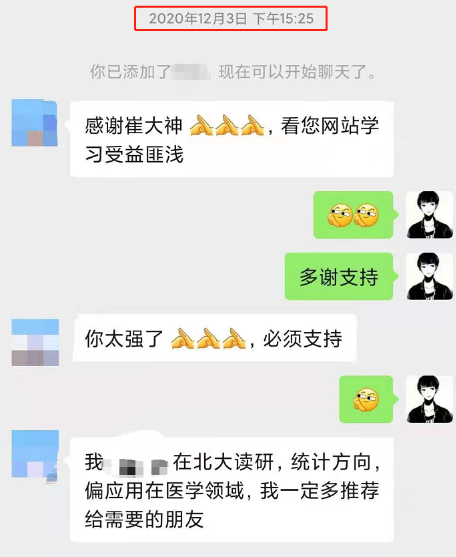去年，123日加了一位读者，简单沟通后，再无过多交流。

直到今年2月春招即将开始，又联系上了我，咨询了一些问题。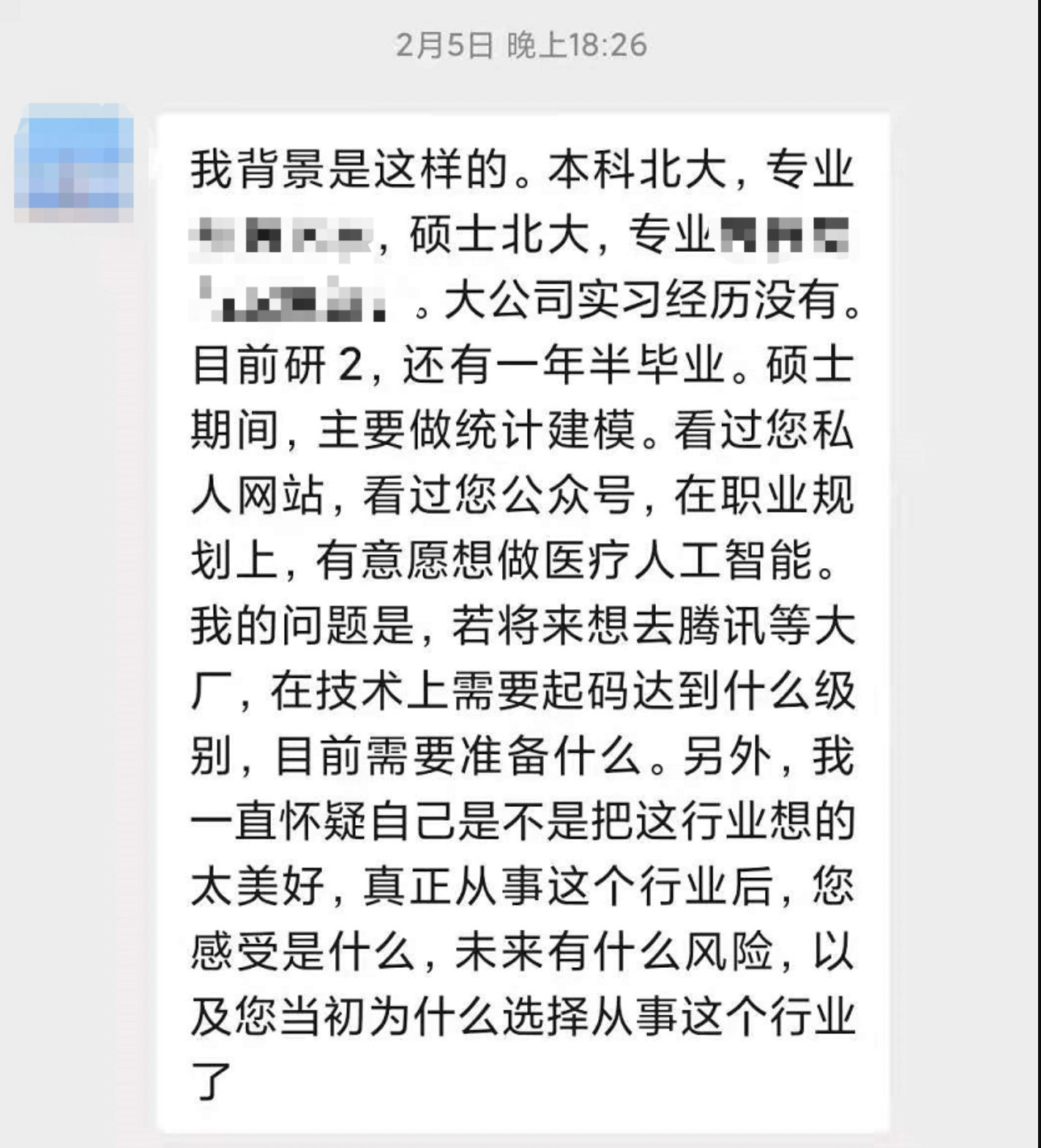看到这些内容，明显的一个感受是，沟通很高效，交代背景+要咨询的问题。

过了几个小时，我有时间了，在回家的路上，做了回复。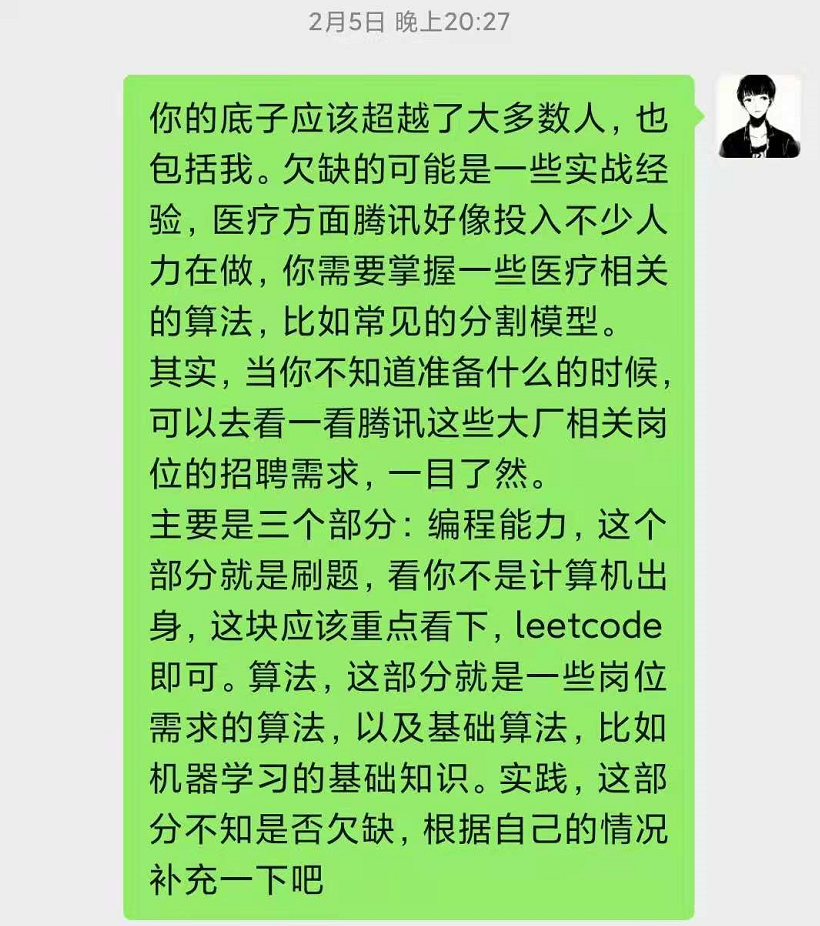然后有不明白的地方，又进行了简单的沟通，比如在Leetcode上刷哪些题之类的，行业现状等等。

然后就在429日，春招即将收官的时候，来报了个喜。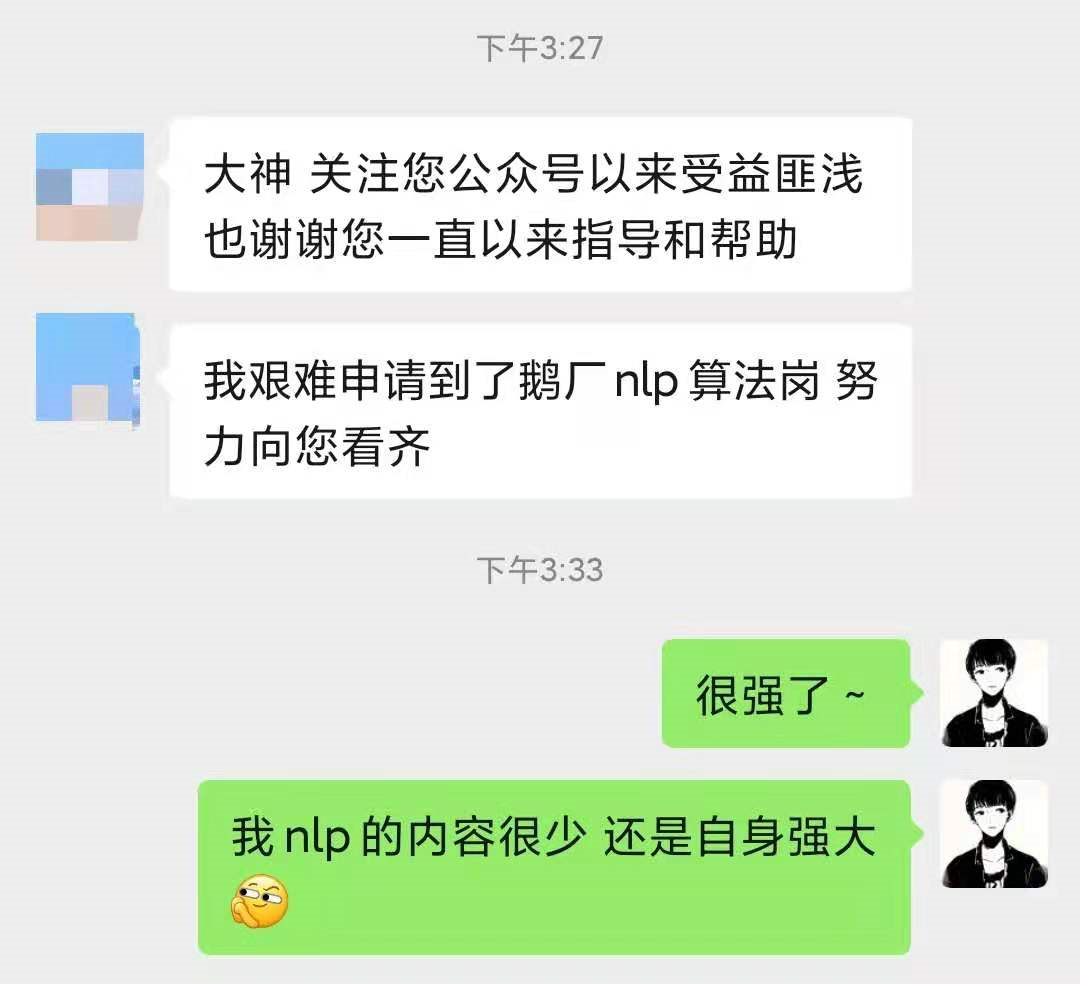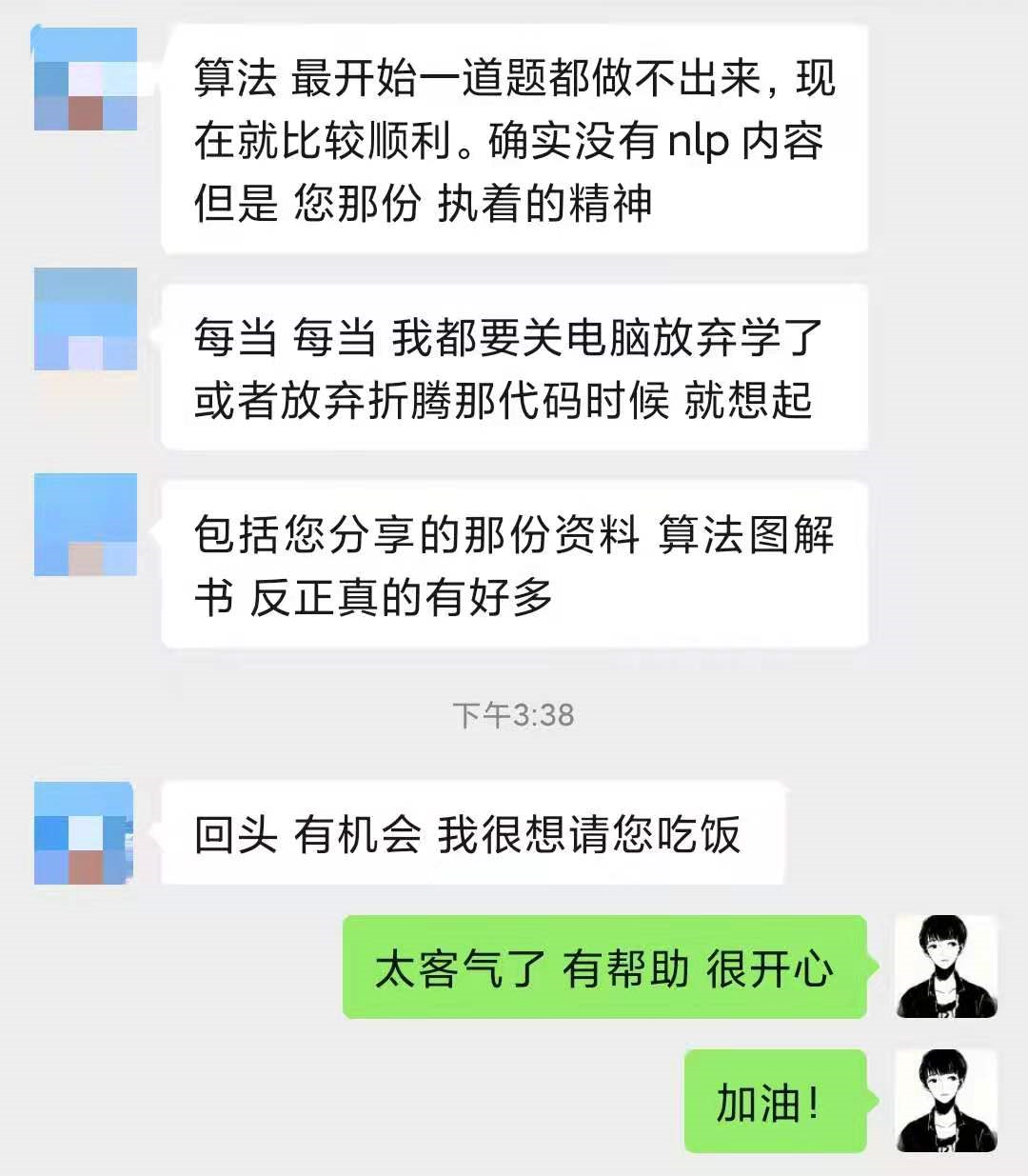顺利进入鹅厂，拿到算法岗实习offer

2月开始题都没有刷过的状态到4月拿到offer高效沟通+高效执行的很好例子。

可能一小部分人会说，北大的光环加持。未曾想，这都是他自己一步一个脚印走出来的，本质上靠的都是坚持不懈的努力。

越优秀的人越努力，一点没错。

其实，我很愿意解答每一个人的问题，分享自己的经验，但前提是建立在良好的沟通上的。

有很小一部分读者，可能不明白这个道理，我曾经也是如此，上来喜欢来句：在吗？有时间吗？

然后其他什么也不说，等我看到的时候，回复了，就没有下文了。

还有的，让我给他写代码，甚至远程帮他调试。时间很充裕的时候，我可能会帮看看代码，但是大多时候，真没这个时间。

看一份陌生的代码，只要不是神，都是一件很费精力的事情

可以试一试，看懂一份别人写的 100 行陌生功能代码，并帮忙找出问题在哪，需要多久？

总之，高效沟通+高效执行，期待我们的共同成长，一起优秀就完事了。

后续，我计划每个月至少出一期高质量的视频，不再给自己找借口，已经断更2个月了，啪啪打脸。

新一期的视频做了一个多星期，每天晚上到家剪辑一些，再写个公众号的文章，时间都是这样挤出来的。但因为视频不露脸，所以需要很多视频素材去转场和衔接，剪辑起来异常耗时。

上次做起来忘了时间，转眼就到了凌晨1点多。水了个读者交流群，就去睡了。

不过这么晚的次数不多，多数时候就到12点，毕竟身体很重要。

接下来，我会继续努力输出技术文，做好自己知识的输入和产出，提高自己的技术，这样也可以带来更多有价值的内容。

当然，五一期间，还想看技术文的读者应该不多，有时间，我就随便写写我的一些日常之类的。

技术文先写着，囤起来，节后再发。

## 结婚那些事

今年，想完成的一件大事，结婚。

今晚，就乘上快乐的小火车，正式地去见家长啦。

纠结了半个月买点什么，最后决定两手空空地去，带着钱包到家再消费。

五一结婚的同学有几个，但因为要去见家长，再加上车票太难买了，只能远程祝福了。

婚后都忙完，还想大胆地折腾一些自己想做的事情。

我本科是做硬件的，过去这么多年了，一直想捡起来。打算买个3D打印机，搭个电脑办公区，硬件焊接区，尽情折腾自己想做的小玩应。

机械+硬件+AI算法，可以做很多有意思的东西，光idea我就想了不少。

再做点视频，争取做个硬核全能up主。

今年要办的事不少，希望大家也都能顺顺利利。

最后再送大家一本帮助我拿到BAT 等一线大厂 offer 的数据结构刷题笔记，是一位 Google 大神写的，对于算法薄弱或者需要提高的同学都十分受用（提起码：m19c）：

BAT 大佬分类总结的 Leetcode 刷题模版，助你搞定 90% 的面试

更加详细的算法学习路线，包括算法、数学、编程语言、比赛等的学习方法和资料，可以看这篇内容：

我是如何成为算法工程师的，超详细的学习路线

最后，喊一句：五一快乐

展开全文• 快乐的深度学习之DQN学习前言简介Q-Learning算法的实现具体实现代码GITHUB下载连接 学习前言 刚刚从大学毕业，近来闲来无事，开始了机器学习的旅程，深度学习是机器学习的重要一环，其可以使得机器自我尝试，并通过...

# 学习前言

刚刚从大学毕业，近来闲来无事，开始了机器学习的旅程，深度学习是机器学习的重要一环，其可以使得机器自我尝试，并通过结果进行学习。在机器学习的过程中，我自网上了解到大神morvanzhou，一个从土木工程转向了计算机的“聪明绝顶”的、英语特好的男人。
morvanzhou的python个人主页，请有兴趣的同学关注大神morvanzhou的python教程。

## 简介

DQN是一种结合了深度学习与强化学习的一种学习方法，它以强化学习Q-Learning为基础，结合tensorflow的神经网络。强化学习Q-Learning是一种非常优秀的算法，但是由于现在许多问题的环境非常复杂，如果把每一种环境都单独列出来，整个Q表会非常大，且更新的时候检索时间较长。所以如果可以不建立Q表，只通过每个环境的特点就可以得出整个环境的Q-Value，那么Q表庞大冗杂的问题就迎刃而解了。
其与常规的强化学习Q-Learning最大的不同就是，DQN在初始化的时候不再生成一个完整的Q-Table，每一个观测环境的Q值都是通过神经网络生成的，即通过输入当前环境的特征Features来得到当前环境每个动作的Q-Value，并且以这个Q-Value基准进行动作选择。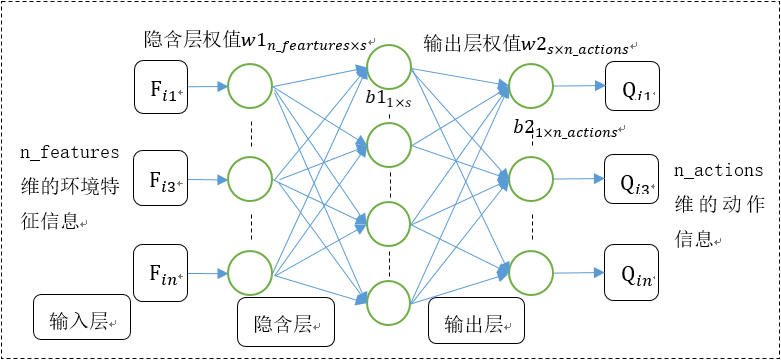DQN一共具有两个神经网络，一个是用于计算q_predict，一个是用于计算q_next的。用于计算q_predict的神经网络，我们简称其为Net_Pre，预测结果被称作Q估计；用于计算q_next我们简称其为Net_Next。预测结果被加工后称作Q现实
DQN的更新准则与Q-Learning的更新准则类似，都是通过所处的当前环境对各个动作的预测得分下一步的环境的实际情况进行得分更新的，但是DQN更新的不再是Q表，而是通过所处的当前环境对各个动作的预测得分下一步的环境的实际情况二者的误差更新Net_Pre和Net_Next的参数
如果大家对Q-Learning还有疑惑，请大家关注我的另一篇博文https://blog.csdn.net/weixin_44791964/article/details/95410737

## DQN算法的实现

接下来我将以小男孩取得玩具为例子，讲述DQN算法的执行过程。在一开始的时候假设小男孩不知道玩具在哪里，Net_Pre和Net_Next都是随机生成的，在进行第一步动作前，小男孩通过Net_Pre获得其对于当前环境每一个动作的得分的预测值，并在其中优先选择得分最高的一个动作作为下一步的行为Action，并且通过该Action得到下一步的环境。

# 刷新环境
env.render()
# DQN 根据观测值选择行为
action = RL.choose_action(observation)
# 环境根据行为给出下一个 state, reward, 是否终止
observation_, reward, done = env.step(action)


在获得所处的当前环境对各个动作的预测得分下一步的环境的实际情况的时候，常规的Q-Learning便可以通过：

self.q_table.loc[observation_now, action] += self.lr * (q_target - q_predict)


进行Q-Table更新，但是DQN不一样，DQN会将这一步按照一定的格式存入记忆，当形成一定规模的时候再进行Net_Pre的更新。在python中，其表示为：

# 控制学习起始时间和频率 (先累积一些记忆再开始学习)
if (step > 200) and (step % 5 == 0):
RL.learn()


可能同学们会很奇怪，为什么是进行Net_Pre的更新而不是进行Net_Pre和Net_Next的更新，其实是因为通过Net_Pre得到的Q_predict的是人物对当前环境的认知，而通过Net_Next通过一定处理之后获得的Q_target是下个环境的实际得分，我们只能通过下个环境的实际得分来更新人物对当前环境的认知
这是否意味着Net_Next不需要更新呢？答案是否定的，因为当前的Net_Next都是随机生成的，其并没有考虑地图上的一个重要信息，就是每个环境的reward。当小男孩掉下悬崖的时候，他的得分是-1；当小男孩拿到玩具的时候，他的得分是1。因此，每当进行一定次数的Net_Pre的更新后，就要将最新的Net_Pre的参数赋值给Net_Next
在python中，其表示为：

if self.learn_step_counter % self.replace_target_iter == 0:
self.sess.run(self.replace_target_op)
print('\ntarget_params_replaced\n')


那么Net_Pre的参数又是如何更新的呢，通过Net_Pre得到的Q_predict的是人物对当前环境的认知，而通过Net_Next通过一定处理之后获得的Q_target是下个环境的实际得分，那么Q_target-Q_predict得到便是实际预测之间的差距，即Q现实Q估计的差距，我们将其作为cost传入tensorflow，便可以使用一定的优化器缩小cost，实现Net_Pre神经网络的训练。接下来让我们看看学习过程是如何实现的。

def learn(self):
# 确认是否到达了需要进行两个神经网络的参数赋值的代数，是则赋值
if self.learn_step_counter % self.replace_target_iter == 0:
self.sess.run(self.replace_target_op)
print('\ntarget_params_replaced\n')

# 根据当前memory当中的size进行提取，在没有到达memory_size时，根据当前的memory里的数量进行提取，提取的数量都是batchsize
if self.memory_counter > self.memory_size:
sample_index = np.random.choice(self.memory_size, size=self.batch_size)
else:
sample_index = np.random.choice(self.memory_counter, size=self.batch_size)

#根据sample_index提取出batch
batch_memory = self.memory[sample_index, :]

#通过神经网络获得q_next和q_eval。
q_next, q_eval = self.sess.run(
[self.q_next, self.q_eval],
feed_dict={
self.s_: batch_memory[:, -self.n_features:],
self.s: batch_memory[:, :self.n_features],
})

#该步主要是为了获得q_next, q_eval的格式
q_target = q_eval.copy()

batch_index = np.arange(self.batch_size, dtype=np.int32)

#取出eval的每一个行为
eval_act_index = batch_memory[:, self.n_features].astype(int)
#取出eval的每一个得分
reward = batch_memory[:, self.n_features + 1]

#取出每一行的最大值、取出每个环境对应的最大得分
q_target[batch_index, eval_act_index] = reward + self.gamma * np.max(q_next, axis=1)

#利用q_target和q_predict训练
_, self.cost = self.sess.run([self._train_op, self.loss],
feed_dict={self.s: batch_memory[:, :self.n_features],
self.q_target: q_target})
self.cost_his.append(self.cost)

#如果存在epsilon_increment则改变epsilon的值
self.epsilon = self.epsilon + self.epsilon_increment if self.epsilon < self.epsilon_max else self.epsilon_max
self.learn_step_counter += 1


可能大家看完这个还是一头雾水，现在，我将以伪代码的方式，将整个DQN执行一遍给大家看一下。

初始化测试环境对象
初始化DQN的大脑对象
step = 0
for episode in range(TIMES):
初始化环境
while(1):
#刷新环境
env.render()
#根据观测值选择行为
action = RL.choose_action(observation)
#获得环境下一步行为，判断下一步环境是否终止, 获得下一步环境的得分。
observation_, reward, done = env.step(action)
#调用存储函数存储记忆
RL.store_transition(observation, action, reward, observation_)
#先累积一些记忆再开始学习
if (step > 200) and (step % 5 == 0):
RL.learn()
#将下一个 state_ 变为 下次循环的 state
#如果终止, 就跳出循环
if done:
break
step += 1   # 总步数


在整个函数的执行过程中DQN的大脑包括以下部分，其对应的功能为

模块名称作用/功能
初始化初始化学习率、可执行动作、全局学习步数、记忆库大小、每次训练的batch大小、两个神经网络等参数
动作选择根据当前所处的环境特征和Net_Pre获得当前环境各个动作的Q_Value，并进行动作选择
学习通过Net_Next获得q_next，再通过一定运算得到q_target，根据q_predict和q_target进行网络更新
记忆存储按照一定格式存储 当前环境特点、下一步环境特点、得分reward、当前环境的动作。

## 具体实现代码

具体的实现代码分为三个部分，这是第一部分，主函数：

from maze_env import Maze
from RL_brain import DeepQNetwork
def run_maze():
step = 0    # 用来控制什么时候学习
for episode in range(300):
# 初始化环境
observation = env.reset()

while True:
# 刷新环境
env.render()

# DQN 根据观测值选择行为
action = RL.choose_action(observation)

# 环境根据行为给出下一个 state, reward, 是否终止
observation_, reward, done = env.step(action)

# DQN 存储记忆
RL.store_transition(observation, action, reward, observation_)

# 控制学习起始时间和频率 (先累积一些记忆再开始学习)
if (step > 200) and (step % 5 == 0):
RL.learn()

# 将下一个 state_ 变为 下次循环的 state
observation = observation_

# 如果终止, 就跳出循环
if done:
break
step += 1   # 总步数

# end of game
print('game over')
env.destroy()

if __name__ == "__main__":
env = Maze()
RL = DeepQNetwork(env.n_actions, env.n_features,
learning_rate=0.01,
reward_decay=0.9,
e_greedy=0.9,
replace_target_iter=200,  # 每 200 步替换一次 target_net 的参数
memory_size=2000, # 记忆上限
# output_graph=True   # 是否输出 tensorboard 文件
)
env.after(100, run_maze)
env.mainloop()
RL.plot_cost()  # 观看神经网络的误差曲线


第二部分是DQN的大脑：

import numpy as np
import pandas as pd
import tensorflow as tf

np.random.seed(1)
tf.set_random_seed(1)

# Deep Q Network off-policy
class DeepQNetwork:
def __init__(
self,
n_actions,
n_features,
learning_rate=0.01,
reward_decay=0.9,
e_greedy=0.9,
replace_target_iter=300,
memory_size=500,
batch_size=32,
e_greedy_increment=None,
output_graph=False,
):
self.n_actions = n_actions      #动作数量
self.n_features = n_features    #观测环境的特征数量
self.lr = learning_rate         #学习率
self.gamma = reward_decay
self.epsilon_max = e_greedy     #处于epsilon范围内时，选择value值最大的动作
self.replace_target_iter = replace_target_iter  #进行参数替换的轮替代数
self.memory_size = memory_size  #每个memory的size
self.batch_size = batch_size    #训练batch的size
self.epsilon_increment = e_greedy_increment
self.epsilon = 0 if e_greedy_increment is not None else self.epsilon_max

self.learn_step_counter = 0     # 初始化全局学习步数

# 初始化用于存储memory的地方 [s, a, r, s_]
self.memory = np.zeros((self.memory_size, n_features * 2 + 2))

# 利用get_collection获得eval和target两个神经网络的参数
self._build_net()
t_params = tf.get_collection('target_net_params')
e_params = tf.get_collection('eval_net_params')
# 将e的参数赋予t
self.replace_target_op = [tf.assign(t, e) for t, e in zip(t_params, e_params)]

self.sess = tf.Session()

#如果output_graph==True则在tensorboard上输出结构
if output_graph:
tf.summary.FileWriter("logs/", self.sess.graph)

self.sess.run(tf.global_variables_initializer())
#用于存储历史cost值
self.cost_his = []

def _build_net(self):
# ------------------ build evaluate_net ------------------
self.s = tf.placeholder(tf.float32, [None, self.n_features], name='s')
#输入值，列的内容为观测到的环境的特点，一共有n个，用于计算对当前环境的估计
self.q_target = tf.placeholder(tf.float32, [None, self.n_actions], name='Q_target')
#该层神经网络用于计算q_eval
with tf.variable_scope('eval_net'):
# 神经网络的参数
c_names =['eval_net_params', tf.GraphKeys.GLOBAL_VARIABLES] #collection的名字
n_l1 = 10   #隐含层神经元的数量
w_initializer = tf.random_normal_initializer(mean = 0., stddev = 0.3)   #初始化正态分布生成器
b_initializer = tf.constant_initializer(0.1)    #常数生成器

# q_eval神经网络的第一层
with tf.variable_scope('l1'):
w1 = tf.get_variable('w1', [self.n_features, n_l1], initializer=w_initializer, collections=c_names)
b1 = tf.get_variable('b1', [1, n_l1], initializer=b_initializer, collections=c_names)
l1 = tf.nn.relu(tf.matmul(self.s, w1) + b1)

# q_eval神经网络的第二层
with tf.variable_scope('l2'):
w2 = tf.get_variable('w2', [n_l1, self.n_actions], initializer=w_initializer, collections=c_names)
b2 = tf.get_variable('b2', [1, self.n_actions], initializer=b_initializer, collections=c_names)
self.q_eval = tf.matmul(l1, w2) + b2

with tf.variable_scope('loss'):     # q_eval神经网络的损失值，其将q_eval的损失值和q_target对比
self.loss = tf.reduce_mean(tf.squared_difference(self.q_target, self.q_eval))
with tf.variable_scope('train'):    # 利用RMSPropOptimizer进行训练
self._train_op = tf.train.RMSPropOptimizer(self.lr).minimize(self.loss)

# ------------------ build target_net ------------------
self.s_ = tf.placeholder(tf.float32, [None, self.n_features], name='s_')    # input
#该层神经网络用于计算q_target
with tf.variable_scope('target_net'):
#神经网络的参数
c_names = ['target_net_params', tf.GraphKeys.GLOBAL_VARIABLES]

#q_target第一层
with tf.variable_scope('l1'):
w1 = tf.get_variable('w1', [self.n_features, n_l1], initializer=w_initializer, collections=c_names)
b1 = tf.get_variable('b1', [1, n_l1], initializer=b_initializer, collections=c_names)
l1 = tf.nn.relu(tf.matmul(self.s_, w1) + b1)

#q_target第二层
with tf.variable_scope('l2'):
w2 = tf.get_variable('w2', [n_l1, self.n_actions], initializer=w_initializer, collections=c_names)
b2 = tf.get_variable('b2', [1, self.n_actions], initializer=b_initializer, collections=c_names)
self.q_next = tf.matmul(l1, w2) + b2

def store_transition(self, s, a, r, s_):
#判断是否有memory_counter属性。没有则加入。该部分用于存储当前状态，action，得分，预测状态。
if not hasattr(self, 'memory_counter'):
self.memory_counter = 0
#将当前状态，action，得分，预测状态进行水平堆叠
transition = np.hstack((s, [a, r], s_))
#index保证在memory_size以内
index = self.memory_counter % self.memory_size
#self.memory中加入一行
self.memory[index, :] = transition
#数量加1
self.memory_counter += 1

def choose_action(self, observation):
#变成可以输入给神经网络的行向量
observation = observation[np.newaxis, :]

if np.random.uniform() < self.epsilon:
#如果在epsilon内，通过运算得出value最大的action，否则随机生成action
actions_value = self.sess.run(self.q_eval, feed_dict={self.s: observation})
action = np.argmax(actions_value)
else:
action = np.random.randint(0, self.n_actions)
return action

def learn(self):
# 确认是否到达了需要进行两个神经网络的参数赋值的代数，是则赋值
if self.learn_step_counter % self.replace_target_iter == 0:
self.sess.run(self.replace_target_op)
print('\ntarget_params_replaced\n')

# 根据当前memory当中的size进行提取，在没有到达memory_size时，根据当前的memory里的数量进行提取，提取的数量都是batchsize
if self.memory_counter > self.memory_size:
sample_index = np.random.choice(self.memory_size, size=self.batch_size)
else:
sample_index = np.random.choice(self.memory_counter, size=self.batch_size)
#根据sample_index提取出batch
batch_memory = self.memory[sample_index, :]

q_next, q_eval = self.sess.run(
[self.q_next, self.q_eval],
feed_dict={
self.s_: batch_memory[:, -self.n_features:],
self.s: batch_memory[:, :self.n_features],
})

q_target = q_eval.copy()

batch_index = np.arange(self.batch_size, dtype=np.int32)

#取出eval的每一个行为
eval_act_index = batch_memory[:, self.n_features].astype(int)
#取出eval的每一个得分
reward = batch_memory[:, self.n_features + 1]

#取出每一行的最大值
q_target[batch_index, eval_act_index] = reward + self.gamma * np.max(q_next, axis=1)

#训练
_, self.cost = self.sess.run([self._train_op, self.loss],
feed_dict={self.s: batch_memory[:, :self.n_features],
self.q_target: q_target})
self.cost_his.append(self.cost)

#如果存在epsilon_increment则改变epsilon的值
self.epsilon = self.epsilon + self.epsilon_increment if self.epsilon < self.epsilon_max else self.epsilon_max
self.learn_step_counter += 1

def plot_cost(self):
import matplotlib.pyplot as plt
plt.plot(np.arange(len(self.cost_his)), self.cost_his)
plt.ylabel('Cost')
plt.xlabel('training steps')
plt.show()


第三部分为运行环境：

"""
Reinforcement learning maze example.
Red rectangle:          explorer.
Black rectangles:       hells       [reward = -1].
Yellow bin circle:      paradise    [reward = +1].
All other states:       ground      [reward = 0].
This script is the environment part of this example.
The RL is in RL_brain.py.
View more on my tutorial page: https://morvanzhou.github.io/tutorials/
"""
import numpy as np
import time
import sys
if sys.version_info.major == 2:
import Tkinter as tk
else:
import tkinter as tk

UNIT = 40   # pixels
MAZE_H = 4  # grid height
MAZE_W = 4  # grid width

class Maze(tk.Tk, object):
def __init__(self):
super(Maze, self).__init__()
self.action_space = ['u', 'd', 'l', 'r']
self.n_actions = len(self.action_space)
self.n_features = 2
self.title('maze')
self.geometry('{0}x{1}'.format(MAZE_H * UNIT, MAZE_H * UNIT))
self._build_maze()

def _build_maze(self):
self.canvas = tk.Canvas(self, bg='white',
height=MAZE_H * UNIT,
width=MAZE_W * UNIT)

# create grids
for c in range(0, MAZE_W * UNIT, UNIT):
x0, y0, x1, y1 = c, 0, c, MAZE_H * UNIT
self.canvas.create_line(x0, y0, x1, y1)
for r in range(0, MAZE_H * UNIT, UNIT):
x0, y0, x1, y1 = 0, r, MAZE_W * UNIT, r
self.canvas.create_line(x0, y0, x1, y1)

# create origin
origin = np.array([20, 20])

# hell
hell1_center = origin + np.array([UNIT * 2, UNIT])
self.hell1 = self.canvas.create_rectangle(
hell1_center - 15, hell1_center - 15,
hell1_center + 15, hell1_center + 15,
fill='black')
# hell
# hell2_center = origin + np.array([UNIT, UNIT * 2])
# self.hell2 = self.canvas.create_rectangle(
#     hell2_center - 15, hell2_center - 15,
#     hell2_center + 15, hell2_center + 15,
#     fill='black')

# create oval
oval_center = origin + UNIT * 2
self.oval = self.canvas.create_oval(
oval_center - 15, oval_center - 15,
oval_center + 15, oval_center + 15,
fill='yellow')

# create red rect
self.rect = self.canvas.create_rectangle(
origin - 15, origin - 15,
origin + 15, origin + 15,
fill='red')

# pack all
self.canvas.pack()

def reset(self):
self.update()
time.sleep(0.1)
self.canvas.delete(self.rect)
origin = np.array([20, 20])
self.rect = self.canvas.create_rectangle(
origin - 15, origin - 15,
origin + 15, origin + 15,
fill='red')
# return observation
return (np.array(self.canvas.coords(self.rect)[:2]) - np.array(self.canvas.coords(self.oval)[:2]))/(MAZE_H*UNIT)

def step(self, action):
s = self.canvas.coords(self.rect)
base_action = np.array([0, 0])
if action == 0:   # up
if s > UNIT:
base_action -= UNIT
elif action == 1:   # down
if s < (MAZE_H - 1) * UNIT:
base_action += UNIT
elif action == 2:   # right
if s < (MAZE_W - 1) * UNIT:
base_action += UNIT
elif action == 3:   # left
if s > UNIT:
base_action -= UNIT

self.canvas.move(self.rect, base_action, base_action)  # move agent

next_coords = self.canvas.coords(self.rect)  # next state

# reward function
if next_coords == self.canvas.coords(self.oval):
reward = 1
done = True
elif next_coords in [self.canvas.coords(self.hell1)]:
reward = -1
done = True
else:
reward = 0
done = False
s_ = (np.array(next_coords[:2]) - np.array(self.canvas.coords(self.oval)[:2]))/(MAZE_H*UNIT)
return s_, reward, done

def render(self):
# time.sleep(0.01)
self.update()


除了环境部分外，主函数部分和DQN大脑部分我都进行了详细的备注，由于涉及到模块间的调用，需要设置文件名称，三个文件的名称分别为：run_this.py，RL_brain.py，maze_env.py。
由于代码并不是自己写的，所以就不上传github了，不过还是欢迎大家关注我和我的github
https://github.com/bubbliiiing/
希望得到朋友们的喜欢。

有不懂的朋友可以评论询问噢。

展开全文人工智能
• 快乐的强化学习6——DDPG及其实现方法学习前言简介举例应用神经网络的构建动作的选择神经网络的学习具体实现代码 学习前言 刚刚从大学毕业，近来闲来无事，开始了机器学习的旅程，深度学习是机器学习的重要一环，其...

# 学习前言

刚刚从大学毕业，近来闲来无事，开始了机器学习的旅程，深度学习是机器学习的重要一环，其可以使得机器自我尝试，并通过结果进行学习。在机器学习的过程中，我自网上了解到大神morvanzhou，一个从土木工程转向了计算机的“聪明绝顶”的、英语特好的男人。
morvanzhou的python个人主页，请有兴趣的同学关注大神morvanzhou的python教程。

## 一、简介

其中：
Deep指的是使用DQN中的经验池和双网络结构来使得神经网络能够有效学习。

如果互联网的广大朋友们希望学习DDPG，一定要明白DQN中Q现实和Q估计的关系，Actor Critic中Actor和Critic之间的关系，大家可以看我其它的博文，有对这两个关系详细的解释。
DQN
Actor Critic

## 二、实现过程拆解

本文使用了OpenAI Gym中的Pendulum-v0游戏来验证算法的实用性。
该环境是一个基于连续动作的环境，其具有一个连续动作。钟摆以随机位置开始，目标是将其向上摆动，使其保持直立。
其不具有终止状态，所以本文设立了进行下一世代的最大STEPS。
其仅具有一个动作，最大值为2，最小值为-2，用于决定其顺时针或者逆时针的用力情况。

### 1、神经网络的构建

对于DDPG而言，其一共需要构建四个神经网络，分别是Actor的现实网络，Actor的估计网络，Critic的现实网络，Critic的估计网络。

#### a、Actor网络部分

在Actor部分，通过输入状态来得到获得一个指定的连续动作。
其建立的神经网络结构如下：
神经网络的输入量是s，指的是环境的状态。
神经网络的输出量是a，指的是一个连续动作的预测情况。

Actor的现实网络接受的输入是s_，代表的是下一环境的状态。
Actor的估计网络接受的输入是s，代表的是当前环境的状态。

对Actor网络进行训练的时候，实际上训练的是Actor的估计网络。其学习过程需要结合critic网络进行学习，其学习过程首先通过当前环境获得当前估计网络预测的动作，再对预测动作进行随机化，将随机化后的动作传入critic网络获得其评分，最后使其评分的value值最大。
Actor部分的神经网络构建函数如下，仅一层，输入值为状态，输出值为连续动作的预测。

# 建立一个actor的层，s为输入量，scope为名称，输出量为-a_bound~a_bound中间的数
def _build_a(self, s, scope, trainable):
with tf.variable_scope(scope):
net = tf.layers.dense(s, 30, activation=tf.nn.relu, name='l1', trainable=trainable)
a = tf.layers.dense(net, self.a_dim, activation=tf.nn.tanh, name='a', trainable=trainable)
# a为-1~1的a_dim维向量
return tf.multiply(a, self.a_bound, name='scaled_a')


如下为actor网络用于学习的损失函数，此处的q为critic网络的输出，在下文会提到：

    # 最大化当前环境的value
a_loss = - tf.reduce_mean(q)    # maximize the q


在实际调用中，分别需要创立两个网络，分别是：
1、用于训练的action估计网络，与DQN的Q估计类似。
2、无需训练的action现实网络，与DQN的Q现实类似。

    # 建立这一步环境和下一步环境的actor网络，
# 这一步环境的actor网络可训练，下一步不可。
# 分别获得两个环境的动作预测结果
with tf.variable_scope('Actor'):
# 共建立两个网络
self.a = self._build_a(self.S, scope='eval', trainable=True)
a_ = self._build_a(self.S_, scope='target', trainable=False)


#### b、Critic网络部分

在Critic部分，通过输入状态和当前的动作来得到该环境下，预测动作的评分。
神经网络的输入量是s，a，指的是环境的状态与对应的动作。
神经网络的输出量是q，指的是该环境下，对应动作的评分value值。

Critic的现实网络接受的输入是s_，a_，代表的是下一环境的状态与动作。
Critic的估计网络接受的输入是s，a，代表的是当前环境的状态与动作。

对Critic网络进行训练的时候，实际上训练的是Critic的估计网络。其学习过程与DQN的Q值更新类似，存在q_target和q_eval。q_target = self.R + GAMMA * q_，代表的是实际的评分。q_eval是当前环境的状态与动作得到的评分，通过训练可以使得估计值越来越接近实际。
如下为critic网络的构建函数，利用a与s共同获得评分value，指的是在环境s下，动作a的评分value值。

# 建立一个critic的层，s、a为输入量，scope为名称
# 该层的输出与状态s和方向a有关，代表的是当处于状态s的时候，选择方向a的价值value
def _build_c(self, s, a, scope, trainable):
with tf.variable_scope(scope):
n_l1 = 30
w1_s = tf.get_variable('w1_s', [self.s_dim, n_l1], trainable=trainable)
w1_a = tf.get_variable('w1_a', [self.a_dim, n_l1], trainable=trainable)
b1 = tf.get_variable('b1', [1, n_l1], trainable=trainable)
net = tf.nn.relu(tf.matmul(s, w1_s) + tf.matmul(a, w1_a) + b1)
return tf.layers.dense(net, 1, trainable=trainable)


如下为critic网络用于学习的损失函数，计算q_eval和q_target的误差平方和，使其最小化：

    # q_target 为当前环境实际评分加上GAMMA*q_，与DQN中相同。
q_target = self.R + GAMMA * q_
# 计算均方误差
td_error = tf.losses.mean_squared_error(labels=q_target, predictions=q)
# 首先训练critic部分，var_list代表被训练的变量


在实际调用中，分别需要创立两个网络，分别是：
1、用于训练的critic预测网络，与DQN的Q估计类似。
2、无需训练的critic实际网络，与DQN的Q现实类似。

    # 建立这一步环境和下一步环境的critic网络，
# 利用这一步环境和这一步的action获得这一步的value估计值
# 这一步环境的critic网络可训练，下一步不可。
# 分别获得两个环境和action的评分预测结果
with tf.variable_scope('Critic'):
# 共建立两个网络
q = self._build_c(self.S, self.a, scope='eval', trainable=True)
q_ = self._build_c(self.S_, a_, scope='target', trainable=False)


#### c、网络连接关系

网络的连接关系为：### 2、动作的选择

Actor的预测网络可以直接输出预测动作，输入量为当前环境的状态。

def choose_action(self, s):
# 本例子进行的是一个连续值的预测，而非离散值。
# self.a是一个shape为[a_dim]的矩阵，a_dim此时为1，因此直接取出
return self.sess.run(self.a, {self.S: s[np.newaxis, :]})


### 3、神经网络的学习

神经网络的学习过程是这样的：
1、进行现实网络与估计网络的软替换，由于现实网络是不会被优化器直接更新的，本文要将估计网络按照一定比例更新到现实网络中。
2、在记忆库中随机获得一定的记忆。
3、对Actor网络进行训练，实际上训练的是Actor的估计网络。其学习过程需要结合critic网络进行学习，其学习过程首先通过当前环境获得当前估计网络预测的动作，再对预测动作进行随机化，将随机化后的动作传入critic网络获得其评分，最后使其评分的value值最大。
4、对Critic网络进行训练，实际上训练的是Critic的估计网络。其学习过程与DQN的Q值更新类似，存在q_target和q_eval。q_target = self.R + GAMMA * q_，代表的是实际的评分。q_eval是当前环境的状态与动作得到的评分，通过训练可以使得估计值越来越接近实际。

def __init__(self, a_dim, s_dim, a_bound,GRAPH_GET =False):
# 记忆库的size
self.memory = np.zeros((MEMORY_CAPACITY, s_dim * 2 + a_dim + 1), dtype=np.float32)
# pointer指向当前记忆所对应的位置，pointer%MEMORY_CAPACITY可以保证index始终在MEMORY_CAPACITY以内。
self.pointer = 0
# 创建会话
self.sess = tf.Session()

self.a_dim, self.s_dim, self.a_bound = a_dim, s_dim, a_bound,
# 当前环境，下一步的环境
self.S = tf.placeholder(tf.float32, [None, s_dim], 's')
self.S_ = tf.placeholder(tf.float32, [None, s_dim], 's_')
# 评分
self.R = tf.placeholder(tf.float32, [None, 1], 'r')

# 建立这一步环境和下一步环境的actor网络，
# 这一步环境的actor网络可训练，下一步不可。
# 分别获得两个环境的动作预测结果
with tf.variable_scope('Actor'):
# 共建立两个网络
self.a = self._build_a(self.S, scope='eval', trainable=True)
a_ = self._build_a(self.S_, scope='target', trainable=False)

# 建立这一步环境和下一步环境的critic网络，
# 利用这一步环境和这一步的action获得这一步的value估计值
# 这一步环境的critic网络可训练，下一步不可。
# 分别获得两个环境和action的评分预测结果
with tf.variable_scope('Critic'):
# 共建立两个网络
q = self._build_c(self.S, self.a, scope='eval', trainable=True)
q_ = self._build_c(self.S_, a_, scope='target', trainable=False)

# 获得每个网络的collection，每个collection是内部变量的集合。
self.ae_params = tf.get_collection(tf.GraphKeys.GLOBAL_VARIABLES, scope='Actor/eval')
self.at_params = tf.get_collection(tf.GraphKeys.GLOBAL_VARIABLES, scope='Actor/target')
self.ce_params = tf.get_collection(tf.GraphKeys.GLOBAL_VARIABLES, scope='Critic/eval')
self.ct_params = tf.get_collection(tf.GraphKeys.GLOBAL_VARIABLES, scope='Critic/target')

# 网络参数的软替换，将当前环境的预测网络模型保留(1-Soft_replace_rate)到下一个环境的预测网络
self.soft_replace = [tf.assign(t, (1 - Soft_replace_rate) * t + Soft_replace_rate * e)
for t, e in zip(self.at_params + self.ct_params, self.ae_params + self.ce_params)]
# q_target 为当前环境实际评分加上GAMMA*q_，与DQN中相同。
q_target = self.R + GAMMA * q_
# 计算均方误差
td_error = tf.losses.mean_squared_error(labels=q_target, predictions=q)
# 首先训练critic部分，var_list代表被训练的变量
# 最大化当前环境的value
a_loss = - tf.reduce_mean(q)    # maximize the q

self.sess.run(tf.global_variables_initializer())
if GRAPH_GET == True:
tf.summary.FileWriter("logs/", self.sess.graph)

def learn(self):
# 每次学习的时候都进行一次软替换
# 将最新训练的结果赋予下一环境的预测组
self.sess.run(self.soft_replace)
# 随机获得一个索引
if self.pointer < MEMORY_CAPACITY:
indices = np.random.choice(self.pointer, size=BATCH_SIZE)
else:
indices = np.random.choice(MEMORY_CAPACITY, size=BATCH_SIZE)
# 取出记忆库中索引对应的一行
bt = self.memory[indices, :]
# 根据记忆前的排序将状态，动作，得分，下一步状态都取出。
bs = bt[:, :self.s_dim]
ba = bt[:, self.s_dim: self.s_dim + self.a_dim]
br = bt[:, -self.s_dim - 1: -self.s_dim]
bs_ = bt[:, -self.s_dim:]

self.sess.run(self.atrain, {self.S: bs})
self.sess.run(self.ctrain, {self.S: bs, self.a: ba, self.R: br, self.S_: bs_})


## 三、具体实现代码

具体的实现代码仅一部分：

import tensorflow as tf
import numpy as np
import gym

MAX_EPISODES = 200  # 最大世代
MAX_EP_STEPS = 200  # 每一时代的最大步数
LR_A = 0.001    # actor学习率
LR_C = 0.002    # critic学习率
GAMMA = 0.9     # 评分衰减率
Soft_replace_rate = 0.01      # 软替换的比率
MEMORY_CAPACITY = 10000 # 记忆容器
BATCH_SIZE = 32 # 每一个batch的size

RENDER_THRESHOLD = -300 # 显示图像刷新的门限
RENDER = False

GRAPH = True    #是否生成tensorboard的图像

env = gym.make('Pendulum-v0')
env = env.unwrapped
env.seed(1)

# 环境的维度
s_dim = env.observation_space.shape
# ACTION的维度
a_dim = env.action_space.shape
# ACTION的范围
a_bound = env.action_space.high
class DDPG(object):
def __init__(self, a_dim, s_dim, a_bound,GRAPH_GET =False):
# 记忆库的size
self.memory = np.zeros((MEMORY_CAPACITY, s_dim * 2 + a_dim + 1), dtype=np.float32)
# pointer指向当前记忆所对应的位置，pointer%MEMORY_CAPACITY可以保证index始终在MEMORY_CAPACITY以内。
self.pointer = 0
# 创建会话
self.sess = tf.Session()

self.a_dim, self.s_dim, self.a_bound = a_dim, s_dim, a_bound,
# 当前环境，下一步的环境
self.S = tf.placeholder(tf.float32, [None, s_dim], 's')
self.S_ = tf.placeholder(tf.float32, [None, s_dim], 's_')
# 评分
self.R = tf.placeholder(tf.float32, [None, 1], 'r')

# 建立这一步环境和下一步环境的actor网络，
# 这一步环境的actor网络可训练，下一步不可。
# 分别获得两个环境的动作预测结果
with tf.variable_scope('Actor'):
# 共建立两个网络
self.a = self._build_a(self.S, scope='eval', trainable=True)
a_ = self._build_a(self.S_, scope='target', trainable=False)

# 建立这一步环境和下一步环境的critic网络，
# 利用这一步环境和这一步的action获得这一步的value估计值
# 这一步环境的critic网络可训练，下一步不可。
# 分别获得两个环境和action的评分预测结果
with tf.variable_scope('Critic'):
# 共建立两个网络
q = self._build_c(self.S, self.a, scope='eval', trainable=True)
q_ = self._build_c(self.S_, a_, scope='target', trainable=False)

# 获得每个网络的collection，每个collection是内部变量的集合。
self.ae_params = tf.get_collection(tf.GraphKeys.GLOBAL_VARIABLES, scope='Actor/eval')
self.at_params = tf.get_collection(tf.GraphKeys.GLOBAL_VARIABLES, scope='Actor/target')
self.ce_params = tf.get_collection(tf.GraphKeys.GLOBAL_VARIABLES, scope='Critic/eval')
self.ct_params = tf.get_collection(tf.GraphKeys.GLOBAL_VARIABLES, scope='Critic/target')

# 网络参数的软替换，将当前环境的预测网络模型保留(1-Soft_replace_rate)到下一个环境的预测网络
self.soft_replace = [tf.assign(t, (1 - Soft_replace_rate) * t + Soft_replace_rate * e)
for t, e in zip(self.at_params + self.ct_params, self.ae_params + self.ce_params)]
# q_target 为当前环境实际评分加上GAMMA*q_，与DQN中相同。
q_target = self.R + GAMMA * q_
# 计算均方误差
td_error = tf.losses.mean_squared_error(labels=q_target, predictions=q)
# 首先训练critic部分，var_list代表被训练的变量
# 最大化当前环境的value
a_loss = - tf.reduce_mean(q)    # maximize the q

self.sess.run(tf.global_variables_initializer())
if GRAPH_GET == True:
tf.summary.FileWriter("logs/", self.sess.graph)

def choose_action(self, s):
# 本例子进行的是一个连续值的预测，而非离散值。
# self.a是一个shape为[a_dim]的矩阵，a_dim此时为1，因此直接取出
return self.sess.run(self.a, {self.S: s[np.newaxis, :]})

def learn(self):
# 每次学习的时候都进行一次软替换
# 将最新训练的结果赋予下一环境的预测组
self.sess.run(self.soft_replace)
# 随机获得一个索引
if self.pointer < MEMORY_CAPACITY:
indices = np.random.choice(self.pointer, size=BATCH_SIZE)
else:
indices = np.random.choice(MEMORY_CAPACITY, size=BATCH_SIZE)
# 取出记忆库中索引对应的一行
bt = self.memory[indices, :]
# 根据记忆前的排序将状态，动作，得分，下一步状态都取出。
bs = bt[:, :self.s_dim]
ba = bt[:, self.s_dim: self.s_dim + self.a_dim]
br = bt[:, -self.s_dim - 1: -self.s_dim]
bs_ = bt[:, -self.s_dim:]

self.sess.run(self.atrain, {self.S: bs})
self.sess.run(self.ctrain, {self.S: bs, self.a: ba, self.R: br, self.S_: bs_})

def store_transition(self, s, a, r, s_):
# 按照状态，动作，得分，下一步状态存入矩阵memory
transition = np.hstack((s, a, [r], s_))
# 状态是在不断替换的
index = self.pointer % MEMORY_CAPACITY
self.memory[index, :] = transition
self.pointer += 1

# 建立一个actor的层，s为输入量，scope为名称，输出量为-a_bound~a_bound中间的数
def _build_a(self, s, scope, trainable):
with tf.variable_scope(scope):
net = tf.layers.dense(s, 30, activation=tf.nn.relu, name='l1', trainable=trainable)
a = tf.layers.dense(net, self.a_dim, activation=tf.nn.tanh, name='a', trainable=trainable)
# a为-1~1的a_dim维向量
return tf.multiply(a, self.a_bound, name='scaled_a')

# 建立一个critic的层，s、a为输入量，scope为名称
# 该层的输出与状态s和方向a有关，代表的是当处于状态s的时候，选择方向a的价值value
def _build_c(self, s, a, scope, trainable):
with tf.variable_scope(scope):
n_l1 = 30
w1_s = tf.get_variable('w1_s', [self.s_dim, n_l1], trainable=trainable)
w1_a = tf.get_variable('w1_a', [self.a_dim, n_l1], trainable=trainable)
b1 = tf.get_variable('b1', [1, n_l1], trainable=trainable)
net = tf.nn.relu(tf.matmul(s, w1_s) + tf.matmul(a, w1_a) + b1)
return tf.layers.dense(net, 1, trainable=trainable)

# 输入量为动作的维度a_dim，环境状态的维度s_dim，动作的最大幅度a_bound
ddpg = DDPG(a_dim, s_dim, a_bound,GRAPH_GET=GRAPH)

# Explore_scope代表在记忆库不够强大时的探索范围
Explore_scope = 3
for i in range(MAX_EPISODES):
s = env.reset()
ep_reward = 0
# 每满200步重新开始一个世代
for j in range(MAX_EP_STEPS):
# RENDER代表是否有图像
if RENDER:
env.render()

# 根据环境选择动作
a = ddpg.choose_action(s)
# 为动作选择增加随机性
a = np.clip(np.random.normal(a, Explore_scope), -2, 2)
# 获取下一步环境特点和分数
s_, r, done, info = env.step(a)
# 按照状态，动作，得分，下一步状态存入矩阵memory
ddpg.store_transition(s, a, r / 10, s_)
# 当记忆库足够大的时候减小Explore_scope值
if ddpg.pointer > MEMORY_CAPACITY:
Explore_scope *= .9995    # decay the action randomness
ddpg.learn()
# 环境更新
s = s_
# 加上当前得分
ep_reward += r
if j == MAX_EP_STEPS-1:
print('Episode:', i, ' Reward: %i' % int(ep_reward), 'Explore_scope: %.2f' % Explore_scope, )
# 当得分大于RENDER_THRESHOLD时，开始显示画面
if ep_reward > RENDER_THRESHOLD:
RENDER = True
break


由于代码并不是自己写的，所以就不上传github了，不过还是欢迎大家关注我和我的github。
https://github.com/bubbliiiing/
希望得到朋友们的喜欢。

有不懂的朋友可以评论询问噢。

展开全文人工智能
• 前阵子有些读者问大学期间的学习路线，说他自己现在有点迷茫。说实话，对于学习路线这种文章，一抓一大堆，也不大喜欢去建议别人究竟该怎么学习学习顺序之类的。不过对于大学，很多人进入大学的时候，可能都是...程序人生
• 学习生活作文500字(通用5篇)导语：在日常学习、工作抑或是生活中，大家都尝试过写作文吧，作文要求篇章结构完整，一定要避免无结尾作文的出现。还是对作文一筹莫展吗？下面是小编为大家整理的学习生活作文...
• 快乐的强化学习5——Actor Critic及其实现方法学习前言简介举例应用神经网络的构建Actor部分Critic部分动作的选择Actor神经网络的学习具体实现代码 学习前言 刚刚从大学毕业，近来闲来无事，开始了机器学习的旅程，...人工智能
• 今天是2020年大年初一，首先祝大家新年快乐。一个人坐在房间，无所事事，于是乎就给大家聊聊学习Java的过程 从大的方面就是三个阶段，分别是Java基础、JavaWeb和Java框架，下面给大家进行个细分。 Java基础 什么...
• 嗯，觉得这个和Java很像 ​ 当重新给字符串变量赋值的时候，变量之前保存的字符串不会被修改，依然在内存中重新给字符串赋值，会重新在内存中开辟空间，这个特点就是字符串的不可变。 ​ ： 由于字符串的不可变，...
• 快乐的深度学习之QLearning学习前言简介Q-Learning算法的实现功能快捷键合理的创建标题，有助于目录的生成如何改变文本的样式插入链接与图片如何插入一段漂亮的代码片生成一个适合你的列表创建一个表格设定内容居中...人工智能
• 快乐的强化学习3——环境模块的调用学习前言gym模块的安装gym模块中环境的常用函数gym的初始化gym的各个参数的获取刷新环境刷新环境应用实例 学习前言 在通常的情况下，手动编写强化学习环境是一件非常耗时间的事情...tensorflow
• 之前有读者问学习计算机基础有什么推荐的书？ 这一个就很有心得了，因为大学的专业并不是计算机专业的，是电气自动化专业的，所以学校的课程并没有操作系统、计算机网络、计算机组成、数据库这类课程，但是...算法
• ## 少儿C++快乐编程

千人学习 2019-08-15 14:49:25
少儿C++快乐编程 适合小学高年级以及中学生自学的编程教程，包含了C++所有的基础知识，用实例教学，在家里就可以学习的少儿编程，不用去价格昂贵的培训机构。每天学习一节课，提高学生的编程能力。c++ c++教程 c++小游戏
• 作者：耿远昊、Datawhale团队 寄语：Pandas 是基于Numpy的一种工具，是为了...Joyful-Pandas（顾名思义：快乐学习Pandas）由Datawhale成员耿远昊发起，作者结合了三份经典教材的学习经验，历时2个多月时间，结合...
•tensorflow
• 全文共2276字，预计学习时长7分钟 图源：Unsplash 进度条真的棒极了！ 进度条直观地展示了一个进程需要多久完成。它使人们不必担心进度停滞，免除了估计代码进程的麻烦。用户可以实时看到程序进程！ ...Python
• C语言编程生日快乐代码的方法：首先要按【CTRL+R】键，cmd进入命令行，输入pip install turtle安装第三方库；然后打开C语言编辑器，将相关代码复制进去；最后按快捷键F5进行保存且执行即可。C语言编程生日快乐代码的...
• ## 机器学习实践应用

万次阅读 多人点赞 2018-04-12 10:04:41
机器学习是人工智能的核心，是使计算机具有 智能的根本途径。 本书通过对机器学习的背景知识、算法流程、相关工具、实践案例以及知识图谱等内容的讲解，全面介绍了机器学习的理论基础和实践应用。书中涉及机器学习...
• 新手学习linux 循序渐进的一个好书。 微博名人推荐。linux 命令行
• python实现生日快乐代码的方法：首先要按【CTRL+R】键，cmd进入命令行，输入pip install turtle安装第三方库；然后打开python IDE，将相关代码复制进去；最后按快捷键F5进行保存且执行即可。python实现生日快乐代码...
• 关注“深度学习自然语言处理”，一起学习一起冲鸭！设为星标，第一时间获取更多干货来源：深度学习与计算机视觉公众号链接：https://www.zhihu.com/question/3057...神经网络 编程语言 人工智能
• 本文将介绍强化学习的基本含义，了解什么是强化学习、强化学习的概念与基本框架以及强化学习中常见的问题类型。 什么是强化学习？ 强化学习（Reinforcement Learning, RL），又称再励学习、评价学习或增强学习，是...算法 数据挖掘
• ## 机器学习笔记一. 特征工程

千次阅读 多人点赞 2022-01-17 09:57:35
\n", transfer.get_feature_names_out()) print("data_new:\n", data_new.toarray()) return None 中文文本特征提取： # 中文文本特征提取：CountVecotrizer def count_demo2(): data = ["我爱学习,学习使我快乐", ...sklearn python
• ## Python 机器学习经典实例

万次阅读 多人点赞 2018-04-12 10:44:15
本书首先通过实用的案例介绍机器学习的基础知识，然后介绍一些稍微复杂的机器学习算法，例如支持向量机、极端随机森林、隐马尔可夫模型、条件随机场、深度神经网络，等等。 用最火的 Python 语言、通过各种各样的...Python
• 作为一名大学生，应该对自己的学习负责，做好大学生的学习计划...为了日后能更好的在社会上立足，要通过在大学期间的学习学到更多的本领，提高自己认识事物判断事物的能力。这是在大学期间的主要目的和任务。：...
• 如果朋友的技术比自己好，还可以趁机学习一下。更重要的是，可以弥补自己的信息差。 02、尽可能扔掉手机 注意啊，可不是真的把手机扔到大街上，你懂的意思，就是让自己远离信息的干扰 。这年头，垃圾的信息太多了...程序员
• ## 程序员如何快乐学习？

千次阅读 多人点赞 2013-07-20 12:04:05
程序员如何学习新的知识？快乐学习的方法？学习的过程？
• 直接上干货、记录此次用自己的云服务器来开启一个深度学习环境搭建初体验、为下一步的 OpenCV炫酷展示打好基础、作为全网 AI 领域 干货最多的博主之一，不负光阴不负卿云服务器使用...